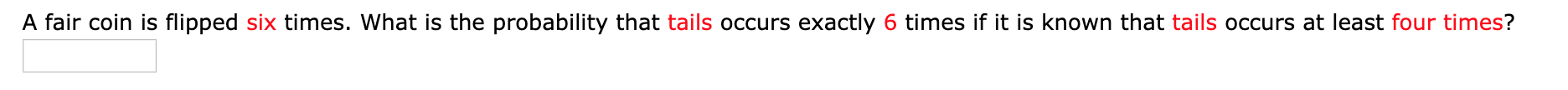# A fair coin is flipped six times. What is the probability that tails occurs exactly 6 times if it is known that tails occurs at least four times?

Question
8 viewshelp_outlineImage TranscriptioncloseA fair coin is flipped six times. What is the probability that tails occurs exactly 6 times if it is known that tails occurs at least four times? fullscreen
check_circle

Step 1

Conditional rule:

The formula for probability of A given B is,

Step 2

In a coin tossing, the probability of getting a head and a tail is equal and the corresponding probability of each is equal to ½. Here,  the coin is tossed 6 times. Thus, it follows a binomial distribution with n=6 and p=1/2. That is,

Step 3

Finding the probability that tails occurs exactly 6 times if it is known that tails occurs at least four times:

The prob...

### Want to see the full answer?

See Solution

#### Want to see this answer and more?

Solutions are written by subject experts who are available 24/7. Questions are typically answered within 1 hour.*

See Solution
*Response times may vary by subject and question.
Tagged in

### Other